## Recent Estimates

### 1. Growth Rates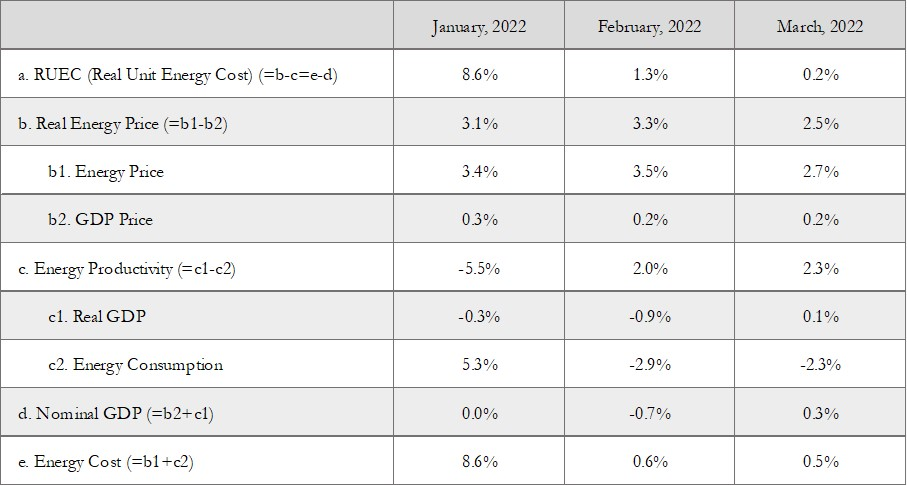Unit: month-over-month growth rates (%). Note: Growth rates are defined as log differences. Notes: The most recent month is the forecasts, the previous month is the preliminary estimates, and the last two months or earlier are the final estimates. The aggregate volume indices of real GDP and energy consumption are measured using the Chained-Laspeyres index, based on the prices and volumes measured at 32 industries and 21 types of energy, respectively. The aggregate price indices of real GDP and energy consumption are defined implicitly.

### 2. IndicesUnit: 2015 average=100. Note: The most recent month is the forecasts, the previous month is the preliminary estimates, and the last two months or earlier are the final estimates.

## Monthly RUEC (Real Unit Energy Cost)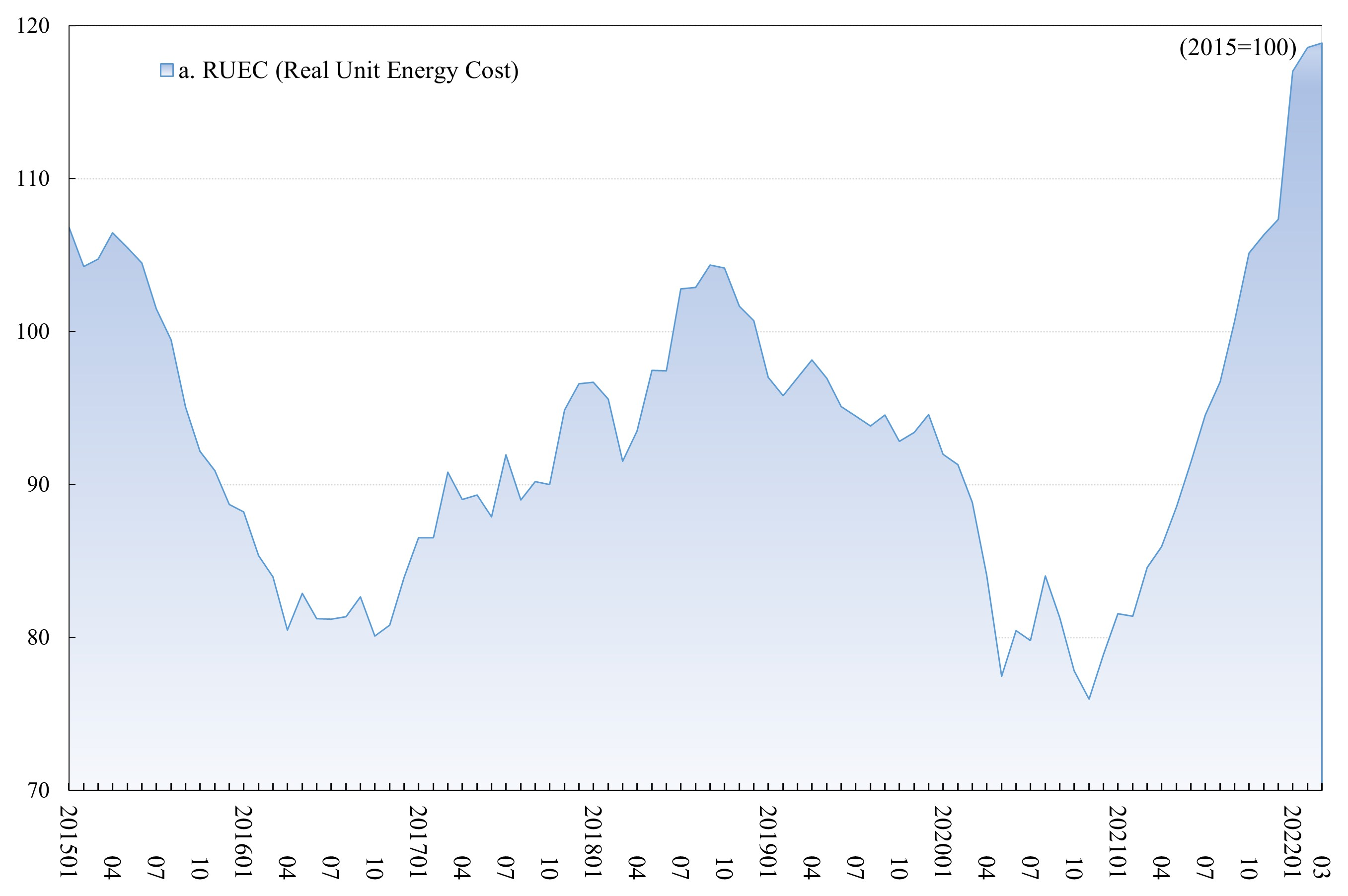Unit: 2015 average=100. Note: Observation period is from January 2015 to March 2022.

## Monthly Real Energy Price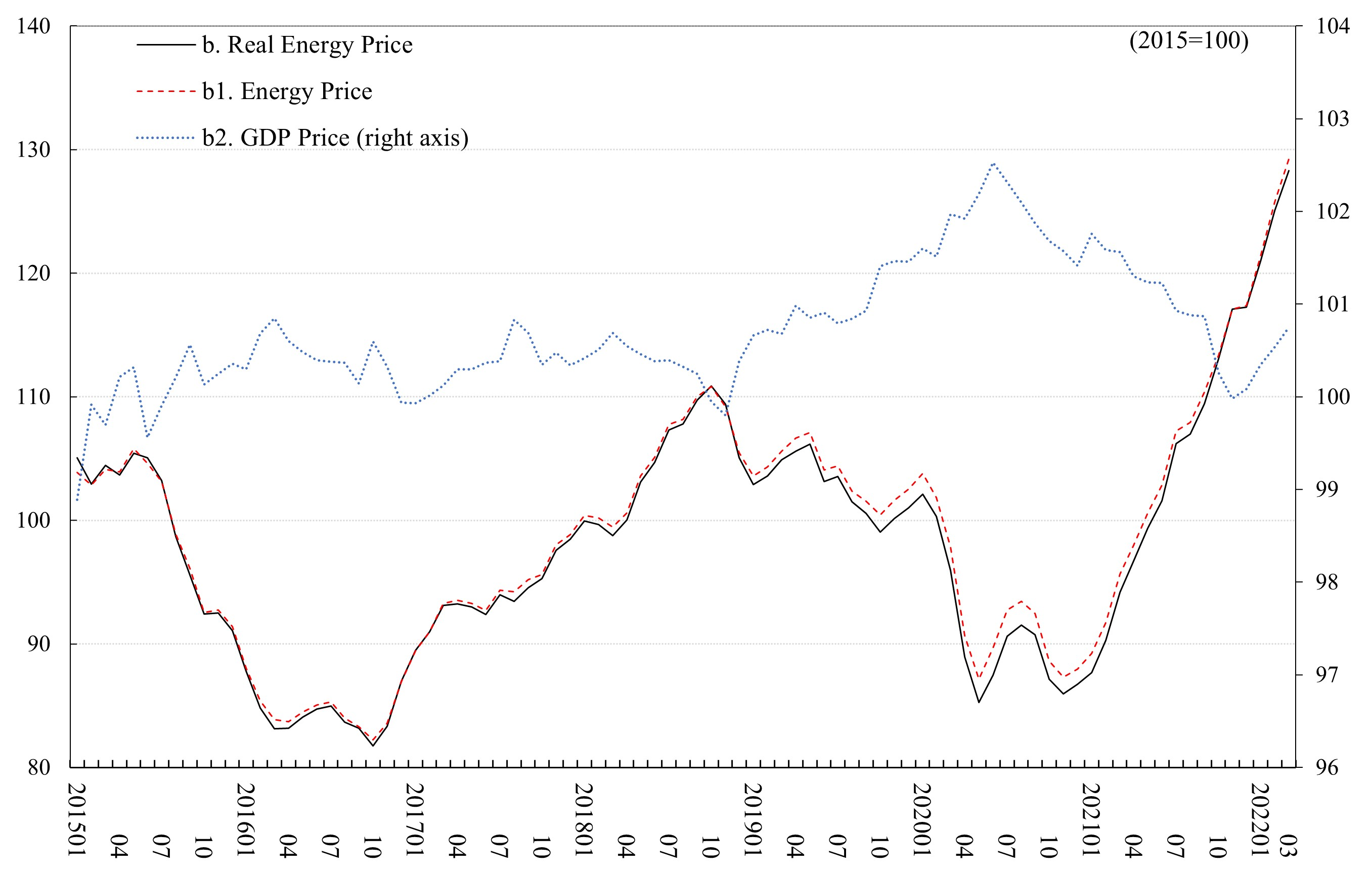Unit: 2015 average=100. Note: Observation period is from January 2015 to March 2022.

## Monthly Real GDP and Energy Productivity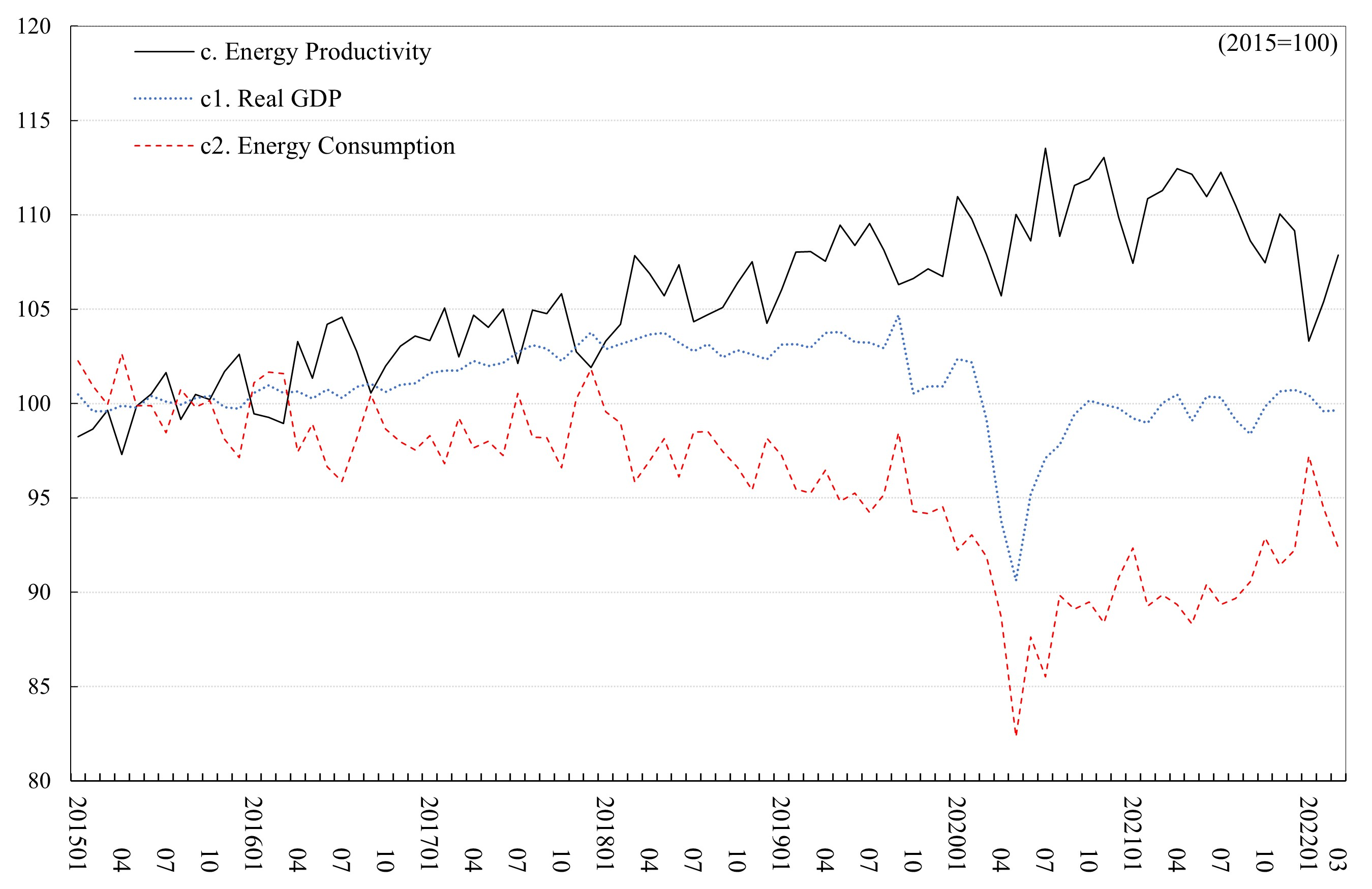Unit: 2015 average=100. Note: Observation period is from January 2015 to March 2022.

## Monthly Nominal GDP and Energy CostUnit: 2015 average=100. Note: Observation period is from January 2015 to March 2022.

## Time-series Data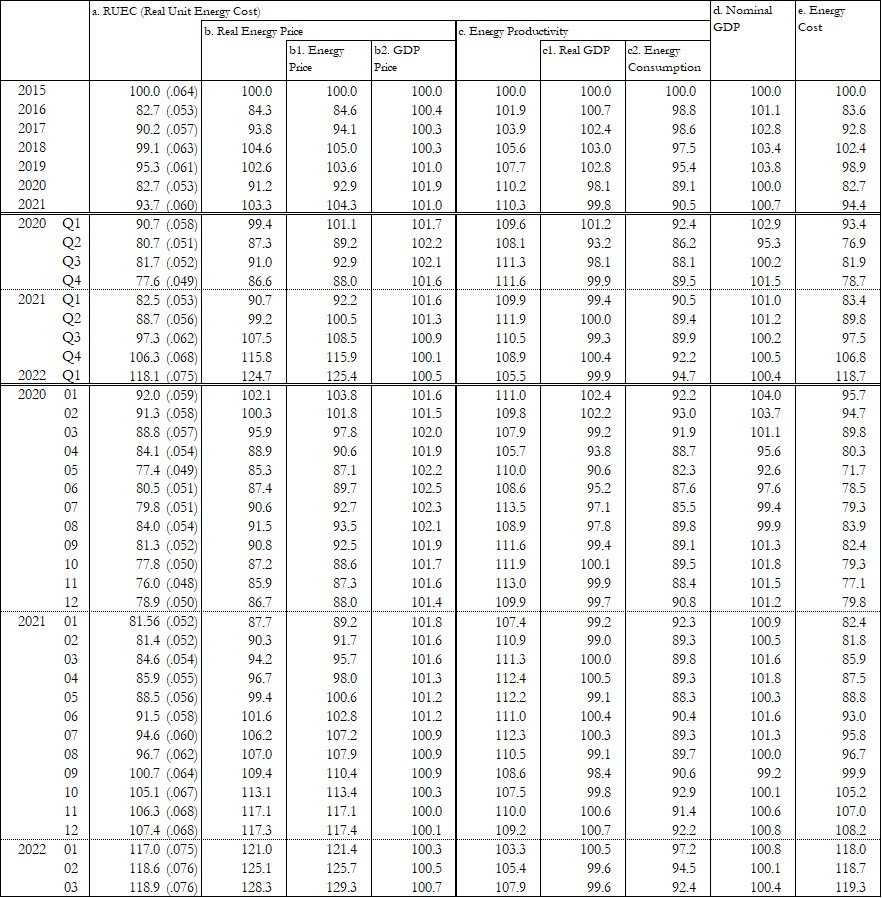Unit: 2015 average=100. Note: The numbers in parentheses are the ratio of final energy consumption cost to GDP at current prices.

## Archive

Data Publication Report Data
ECM_JPN_202205 To be published on May 31 n.a. n.a.
ECM_JPN_202204 April 30, 2022 [pdf] [excel]
ECM_JPN_202203 April 12, 2022 [pdf] [excel]